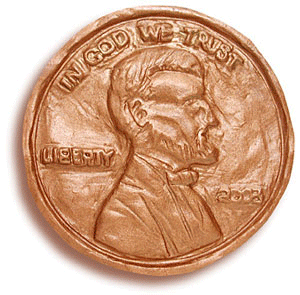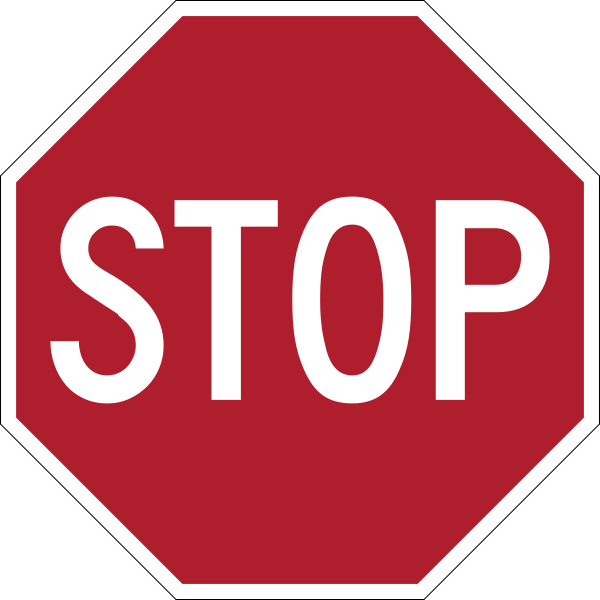## Simulating Coin TossesSo now we want to use the coin tosses to model the "just guessing" choices people make between the two faces. Using coin tosses is valid because we are assuming each and every person was equally likely to put Tim on the left (=Heads) or Bob (=Tails).

Parallels between real study and simulation

 Coin flip Choice between Bob and Tim Heads Tim on left Tails Bob on left Chance of heads 0.50 One repetition One set of %n1% coin tosses

Scroll down to see further instructions for using the applet.

(f) What are the observational units and variable in this dotplot? [Hints: You should have 10 dots. What "entity" does each dot represent and what "question" was measured for each dot? What would you have to do to add another dot - this is your observational unit!]The previous question is pretty important, ASK if you are not sure.

(g) Is the variable you identified for the dotplot in (f) quantitative or categorical?